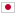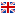I - Revenge of the Endless BFS/Problem Statement

Mr. Endo wanted to write the code that performs breadth-first search (BFS), which is a search algorithm to explore all vertices on a directed graph. An example of pseudo code of BFS is as follows:

1: $current \leftarrow \{start\_vertex\}$ 2: $visited \leftarrow current$ 3: while $visited \ne$ the set of all the vertices 4: $found \leftarrow \{\}$ 5: for $u$ in $current$ 6: for each $v$ such that there is an edge from $u$ to $v$ 7: $found \leftarrow found \cup \{v\}$ 8: $current \leftarrow found \setminus visited$ 9: $visited \leftarrow visited \cup found$

However, Mr. Endo apparently forgot to manage visited vertices in his code. More precisely, he wrote the following code:

1: $current \leftarrow \{start\_vertex\}$ 2: while $current \ne$ the set of all the vertices 3: $found \leftarrow \{\}$ 4: for $u$ in $current$ 5: for each $v$ such that there is an edge from $u$ to $v$ 6: $found \leftarrow found \cup \{v\}$ 7: $current \leftarrow found$

You may notice that for some graphs, Mr. Endo's program will not stop because it keeps running infinitely. Notice that it does not necessarily mean the program cannot explore all the vertices within finite steps. Your task here is to make a program that determines whether Mr. Endo's program will stop within finite steps for a given directed graph in order to point out the bug to him. Also, calculate the minimum number of loop iterations required for the program to stop if it is finite. Since the answer might be huge, thus print the answer modulo $10^9 + 7$, which is a prime number.

Input

The input consists of a single test case formatted as follows.

$N$ $M$ $u_{1}$ $v_{1}$ $\vdots$ $u_{M}$ $v_{M}$

The first line consists of two integers $N$ ($2 \le N \le 500$) and $M$ ($1 \le M \le 200{,}000$), where $N$ is the number of vertices and $M$ is the number of edges in a given directed graph, respectively. The $i$-th line of the following $M$ lines consists of two integers $u_{i}$ and $v_{i}$ ($1 \le u_{i}, v_{i} \le N$), which means there is an edge from $u_{i}$ to $v_{i}$ in the given graph. The vertex $1$ is the start vertex, i.e. $start\_vertex$ in the pseudo codes. You can assume that the given graph also meets the following conditions.

• The graph has no self-loop, i.e., $u_{i} \ne v_{i}$ for all $1 \le i \le M$.
• The graph has no multi-edge, i.e., $(u_{i}, v_{i}) \ne (u_{j}, v_{j})$ for all $1 \le i < j \le M$.
• For each vertex $v$, there is at least one path from the start vertex $1$ to $v$.

Output

If Mr. Endo's wrong BFS code cannot stop within finite steps for the given input directed graph, print -1 in a line. Otherwise, print the minimum number of loop iterations required to stop modulo $10^9 + 7$.

4 4
1 2
2 3
3 4
4 1

-1

4 5
1 2
2 3
3 4
4 1
1 3

7

5 13
4 2
2 4
1 2
5 4
5 1
2 1
5 3
4 3
1 5
4 5
2 3
5 2
1 3

3